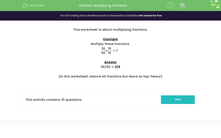# Multiply Fractions

In this worksheet, students will practise multiplying fractions.Key stage:  KS 3

Curriculum topic:   Number

Curriculum subtopic:   Use Four Operations for All Numbers

Difficulty level:#### Worksheet Overview

This activity is about multiplying fractions.

Example

Multiply these fractions.

 4 x 2 5 8

 4 x 2 = 8 5 x 8 40

Remember to simplify fractions if they have a common factor.

In this example, the highest common factor of 8 and 40 is 8 so we can divide both numerator and denominator by 8.

8/40 = 1/5

In this activity, we need to reduce all fractions to their simplified form but leave them as top-heavy, or improper.

### What is EdPlace?

We're your National Curriculum aligned online education content provider helping each child succeed in English, maths and science from year 1 to GCSE. With an EdPlace account you’ll be able to track and measure progress, helping each child achieve their best. We build confidence and attainment by personalising each child’s learning at a level that suits them.

Get started